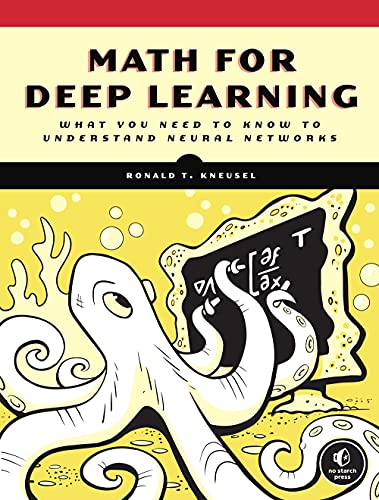# Math for Deep Learning: What You Need to Know to Understand Neural Networks

• Length: 344 pages
• Edition: 1
• Publisher:
• Publication Date: 2021-11-23
• ISBN-10: 1718501900
• ISBN-13: 9781718501904
Description

Math for Deep Learning provides the essential math you need to understand deep learning discussions, explore more complex implementations, and better use the deep learning toolkits.

With Math for Deep Learning, you’ll learn the essential mathematics used by and as a background for deep learning.

You’ll work through Python examples to learn key deep learning related topics in probability, statistics, linear algebra, differential calculus, and matrix calculus as well as how to implement data flow in a neural network, backpropagation, and gradient descent. You’ll also use Python to work through the mathematics that underlies those algorithms and even build a fully-functional neural network.